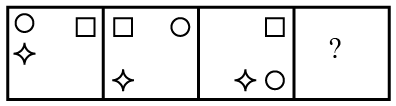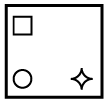Question 2

# Select the figure that can replace the question mark (?) in the following series.Solution

In the given series, the circle is moving one place in clockwise direction along the corners of the square. The star symbol is moving one place in anticlockwise direction along the sides and corners alternatively. The square is moving one place front and back alternatively.

$$\therefore\$$The next figure in the series isHence, the correct answer is Option A